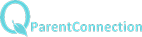Statistics and Probability

FRQ

QuestionsChapter 14

Outline

Java Applets

Section 14.1

1.    Conditions for inference
a.    The response variable varies according to a

normal distribution.
b.    The mean response (mu of y) has a straight-

line relationship with the explanatory

variable x.
c.    The standard deviation for the response

variable is the same for all values for x.
2.    Inference
a.    Estimators for the slope and intercept
b.    The standard error of the line is based upon

the residuals.
i.    Degree of Freedom is n-2
3.    Confidence Interval
a.    Estimation for the regression slope.
4.    Hypothesis testing for the slope
a.    The null hypothesis will always be no relation
b.    The alternative will be concerning positive or

negative slope.  If the alternative is “not

equal” to zero, then the statement is that
there is some relationship between the x and

the y variable.

Section 14.2

A.    Confidence interval for average response
1.    This is the confidence interval based upon the

least squares regression model.
B.    Confidence interval for single observation
1.    Prediction for an observation based upon an

individual response.

AP Statistics

Syllabus

Buchanan High School     |     1560 N. Minnewawa Ave.    |     Clovis, CA     |     93619     |     (559) 327-3000